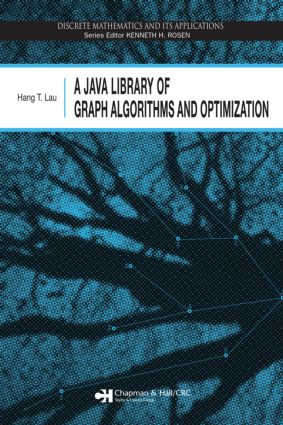# A Java Library of Graph Algorithms and Optimization

## 1st Edition

Chapman and Hall/CRC

386 pages

##### Purchasing Options:\$ = USD
Hardback: 9781584887188
pub: 2006-10-20
\$145.00
x
eBook (VitalSource) : 9780429140815
pub: 2006-10-20
from \$28.98

FREE Standard Shipping!

### Description

Because of its portability and platform-independence, Java is the ideal computer programming language to use when working on graph algorithms and other mathematical programming problems. Collecting some of the most popular graph algorithms and optimization procedures, A Java Library of Graph Algorithms and Optimization provides the source code for a library of Java programs that can be used to solve problems in graph theory and combinatorial optimization. Self-contained and largely independent, each topic starts with a problem description and an outline of the solution procedure, followed by its parameter list specification, source code, and a test example that illustrates the usage of the code.

The book begins with a chapter on random graph generation that examines bipartite, regular, connected, Hamilton, and isomorphic graphs as well as spanning, labeled, and unlabeled rooted trees. It then discusses connectivity procedures, followed by a paths and cycles chapter that contains the Chinese postman and traveling salesman problems, Euler and Hamilton cycles, and shortest paths. The author proceeds to describe two test procedures involving planarity and graph isomorphism. Subsequent chapters deal with graph coloring, graph matching, network flow, and packing and covering, including the assignment, bottleneck assignment, quadratic assignment, multiple knapsack, set covering, and set partitioning problems. The final chapters explore linear, integer, and quadratic programming. The appendices provide references that offer further details of the algorithms and include the definitions of many graph theory terms used in the book.

INTRODUCTION

RANDOM GRAPH GENERATION

Random Permutation of n Objects

Random Graph

Random Bipartite Graph

Random Regular Graph

Random Spanning Tree

Random Labeled Tree

Random Unlabeled Rooted Tree

Random Connected Graph

Random Hamilton Graph

Random Maximum Flow Network

Random Isomorphic Graphs

Random Isomorphic Regular Graphs

CONNECTIVITY

Maximum Connectivity

Depth-First Search

Connected Graph Testing

Connected Components

Cut Nodes

Strongly Connected Components

Minimal Equivalent Graph

Edge Connectivity

Minimum Spanning Tree

All Cliques

PATHS AND CYCLES

Fundamental Set of Cycles

Shortest Cycle Length

One-Pair Shortest Path

All Shortest Path Length

Shortest Path Tree

All Pairs Shortest Paths

k Shortest Paths

k Shortest Paths without Repeated Nodes

Euler Circuit

Hamilton Cycle

Chinese Postman Tour

Traveling Salesman Problem

PLANARITY TESTING

GRAPH ISOMORPHISM TESTING

COLORING

Node Coloring

Chromatic Polynomial

GRAPH MATCHING

Maximum Cardinality Matching

Minimum Sum Perfect Matching

NETWORK FLOW

Maximum Network Flow

Minimum Cost Network Flow

PACKING AND COVERING

Assignment Problem

Bottleneck Assignment Problem

Multiple Knapsack Problem

Set Covering Problem

Set Partitioning Problem

LINEAR PROGRAMMING

Revised Simplex Method

Dual Simplex Method

INTEGER PROGRAMMING

Zero-One Integer Programming

All Integer Programming

Mixed Integer Programming

APPENDIX A: REFERENCES

APPENDIX B: GRAPH-THEORETIC TERMS

INDEX OF PROCEDURES

### Subject Categories

##### BISAC Subject Codes/Headings:
COM051300
COMPUTERS / Programming / Algorithms
MAT000000
MATHEMATICS / General
MAT021000
MATHEMATICS / Number Systems